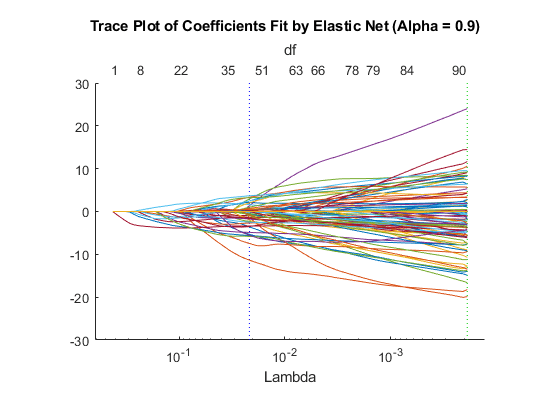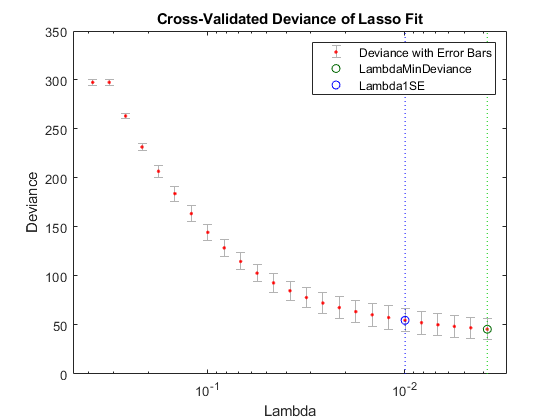# Regularize Wide Data in Parallel

This example shows how to regularize a model with many more predictors than observations. Wide data is data with more predictors than observations. Typically, with wide data you want to identify important predictors. Use `lassoglm` as an exploratory or screening tool to select a smaller set of variables to prioritize your modeling and research. Use parallel computing to speed up cross validation.

Load the `ovariancancer` data. This data has 216 observations and 4000 predictors in the `obs` workspace variable. The responses are binary, either `'Cancer'` or `'Normal'`, in the `grp` workspace variable. Convert the responses to binary for use in `lassoglm`.

```load ovariancancer y = strcmp(grp,'Cancer');```

Set options to use parallel computing. Prepare to compute in parallel using `parpool`.

```opt = statset('UseParallel',true); parpool()```
```Starting parallel pool (parpool) using the 'local' profile ... connected to 6 workers. ans = Pool with properties: Connected: true NumWorkers: 6 Cluster: local AttachedFiles: {} AutoAddClientPath: true IdleTimeout: 30 minutes (30 minutes remaining) SpmdEnabled: true ```

Fit a cross-validated set of regularized models. Use the `Alpha` parameter to favor retaining groups of highly correlated predictors, as opposed to eliminating all but one member of the group. Commonly, you use a relatively large value of `Alpha`.

```rng('default') % For reproducibility tic [B,S] = lassoglm(obs,y,'binomial','NumLambda',100, ... 'Alpha',0.9,'LambdaRatio',1e-4,'CV',10,'Options',opt); toc```
```Elapsed time is 82.390258 seconds. ```

Examine cross-validation plot.

```lassoPlot(B,S,'PlotType','CV'); legend('show') % Show legend```Examine trace plot.

`lassoPlot(B,S,'PlotType','Lambda','XScale','log')`The right (green) vertical dashed line represents the `Lambda` providing the smallest cross-validated deviance. The left (blue) dashed line has the minimal deviance plus no more than one standard deviation. This blue line has many fewer predictors:

`[S.DF(S.Index1SE) S.DF(S.IndexMinDeviance)]`
```ans = 1×2 50 89 ```

You asked `lassoglm` to fit using 100 different `Lambda` values. How many did it use?

`size(B)`
```ans = 1×2 4000 84 ```

`lassoglm` stopped after 84 values because the deviance was too small for small `Lambda` values. To avoid overfitting, `lassoglm` halts when the deviance of the fitted model is too small compared to the deviance in the binary responses, ignoring the predictor variables.

You can force `lassoglm` to include more terms by using the `'Lambda'` name-value pair argument. For example, define a set of `Lambda` values that additionally includes three values smaller than the values in `S.Lambda`.

```minLambda = min(S.Lambda); explicitLambda = [minLambda*[.1 .01 .001] S.Lambda];```

Specify `'Lambda',explicitLambda` when you call the `lassoglm` function. `lassoglm` halts when the deviance of the fitted model is too small, even though you explicitly provide a set of `Lambda` values.

To save time, you can use:

• Fewer `Lambda`, meaning fewer fits

• Fewer cross-validation folds

• A larger value for `LambdaRatio`

Use serial computation and all three of these time-saving methods:

```tic [Bquick,Squick] = lassoglm(obs,y,'binomial','NumLambda',25,... 'LambdaRatio',1e-2,'CV',5); toc```
```Elapsed time is 14.668383 seconds. ```

Graphically compare the new results to the first results.

```lassoPlot(Bquick,Squick,'PlotType','CV'); legend('show') % Show legend````lassoPlot(Bquick,Squick,'PlotType','Lambda','XScale','log')`The number of nonzero coefficients in the lowest plus one standard deviation model is around 50, similar to the first computation.